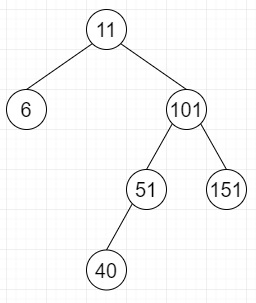# Check if a given Binary Tree is height balanced like a Red-Black Tree in Python

Suppose there is a Red-Black Tree, here the largest height of a node is at most double the minimum height. If we have a binary search tree, we have to check the following property. With respect of every node, length of the longest leaf to node path has not more than double the nodes on shortest path from node to leaf.

So, if the input is likethen the output will be True, as this is balanced.

To solve this, we will follow these steps −

• Define a function solve() . This will take root, max_height, min_height
• if root is null, then
• max_height := 0, min_height := 0
• return True
• left_max := 0, left_min := 0
• right_max := 0, right_min := 0
• if solve(root.left, left_max, left_min) is same as False, then
• return False
• if solve(root.right, right_max, right_min) is same as False, then
• return False
• max_height := maximum of left_max and right_max + 1
• min_height := minimum of left_min and right_min + 1
• if max_height <= 2 * min_height, then
• return True
• return False
• From the main method do the following −
• max_height := 0, min_height := 0
• return solve(root, max_height, min_height)

## Example

Let us see the following implementation to get better understanding −

Live Demo

class TreeNode:
def __init__(self, key):
self.data = key
self.left = None
self.right = None
def solve(root, max_height, min_height) :
if (root == None) :
max_height = min_height = 0
return True
left_max=0
left_min=0
right_max, right_min=0,0
if (solve(root.left, left_max, left_min) == False) :
return False
if (solve(root.right, right_max, right_min) == False) :
return False
max_height = max(left_max, right_max) + 1
min_height = min(left_min, right_min) + 1
if (max_height <= 2 * min_height) :
return True
return False
def is_tree_balanced(root) :
max_height, min_height = 0,0
return solve(root, max_height, min_height)
root = TreeNode(10)
root.left = TreeNode(5)
root.right = TreeNode(100)
root.right.left = TreeNode(50)
root.right.right = TreeNode(150)
root.right.left.left = TreeNode(40)
print(is_tree_balanced(root))

## Input

root = TreeNode(10)
root.left = TreeNode(5)
root.right = TreeNode(100)
root.right.left = TreeNode(50)
root.right.right = TreeNode(150)
root.right.left.left = TreeNode(40)

## Output

True